# Math in Focus Grade 5 Chapter 4 Practice 4 Answer Key Multiplying Mixed Numbers and Whole Numbers

Go through the Math in Focus Grade 5 Workbook Answer Key Chapter 4 Practice 4 Multiplying Mixed Numbers and Whole Numbers to finish your assignments.

## Math in Focus Grade 5 Chapter 4 Practice 4 Answer Key Multiplying Mixed Numbers and Whole Numbers

Complete.

Question 1.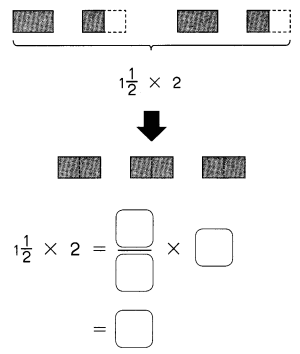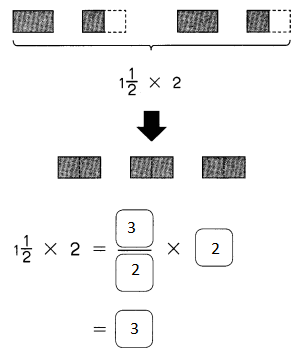Explanation:
Simplified the mixed fraction and multiplied.

Question 2.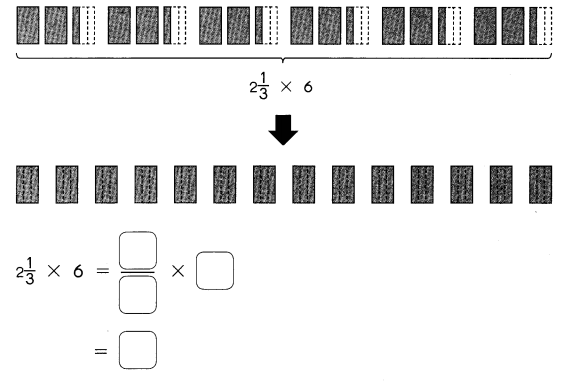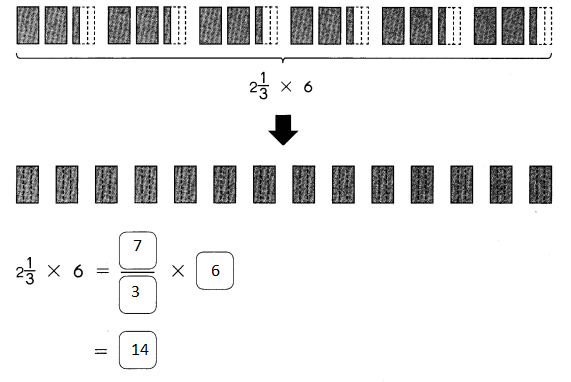Explanation:
Simplified the mixed fraction  and multiplied.

Multiply. Express the product as a whole number or a mixed number in simplest form.

Example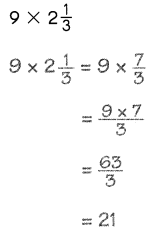Question 3.
4$$\frac{1}{5}$$ × 15 =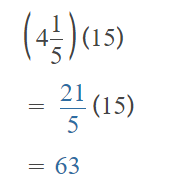Explanation:
Simplified the mixed fraction and multiplied. simplified to whole number.

Question 4.
2$$\frac{3}{7}$$ × 28 =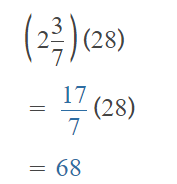Explanation:
Simplified the mixed fraction and multiplied. simplified to whole number.

Question 5.
24 × 1$$\frac{5}{6}$$ =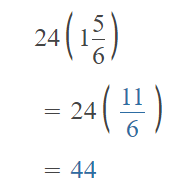Explanation:
Simplified the mixed fraction and multiplied. simplified to whole number.

Question 7.
4$$\frac{1}{2}$$ × 18 =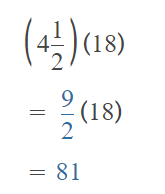Explanation:
Simplified the mixed fraction and multiplied. simplified to whole number.

Multiply. Express the product as a whole number or a mixed number in simplest form.

Question 7.
2$$\frac{3}{4}$$ × 16 =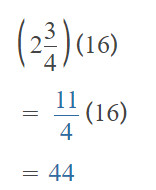Explanation:
Simplified the mixed fraction and multiplied. simplified to whole number.

Question 8.
32 × 3$$\frac{1}{8}$$ =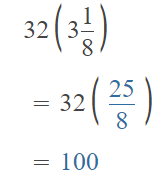Explanation:
Simplified the mixed fraction and multiplied. simplified to whole number.

Multiply. Express the product as a whole number or a mixed number in simplest form.

Example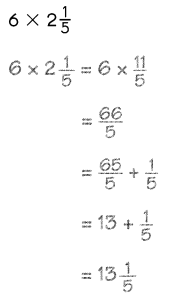Question 9.
4 × 2$$\frac{7}{9}$$ =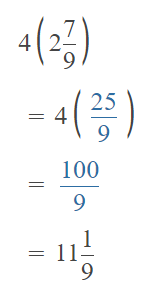Explanation:
Simplified the mixed fraction and multiplied. simplified to mixed fraction .

Question 10.
5 × 2$$\frac{3}{7}$$ =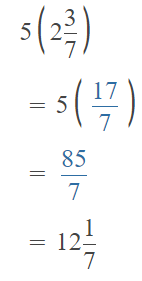Explanation:
Simplified the mixed fraction and multiplied. simplified to mixed fraction .

Multiply. Express the product as a whole number or a mixed number in simplest form.

Question 11.
2$$\frac{1}{4}$$ × 7 =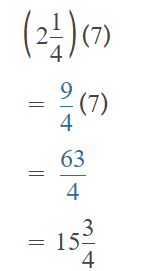Explanation:
Simplified the mixed fraction and multiplied. simplified to mixed fraction .

Question 12.
1$$\frac{4}{5}$$ × 12 =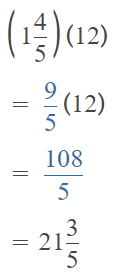Explanation:
Simplified the mixed fraction and multiplied. simplified to mixed fraction .

Question 13.
12 × 2$$\frac{3}{8}$$ =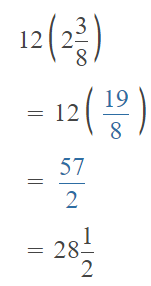Explanation:
Simplified the mixed fraction and multiplied. simplified to mixed fraction .

Question 14.
26 × 1$$\frac{1}{6}$$ =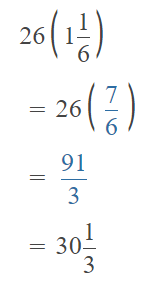Explanation:
Simplified the mixed fraction and multiplied. simplified to mixed fraction .

Question 15.
Is the product of 6 and 10 greater than or less than each of its factors? Explain your reasoning.
6 x 10 = 60
Factors of 6 are 2, 3, 6, 1
Factors of 10 are 1, 2, 5, and 10.
Explanation:
The factors are lesser and equal to the product of 6 and 10

Question 16.
Is the product of $$\frac{2}{5}$$ and 5 greater than or less than $$\frac{2}{5}$$? Is it greater than or less than 5? Explain your reasoning.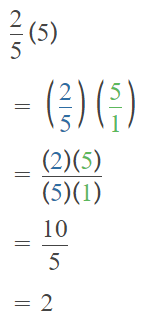The product of $$\frac{2}{5}$$ and 5 is greater than $$\frac{2}{5}$$
The product of $$\frac{2}{5}$$ and 5 is less than 5